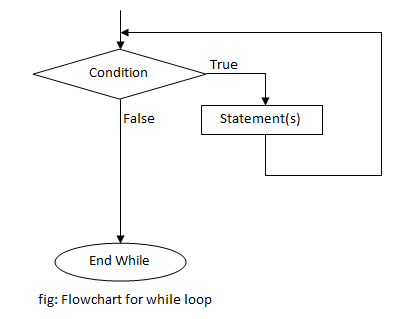# while loop in C++ Programming

In every programming language including C++, loop is a process of repeating a group of statements until a certain condition is satisfied. While loop is an entry controlled loop where the condition is checked at the beginning of the loop. The condition to be checked can be changed inside it. The control can exit a loop in two ways, when the condition becomes false or using break statement.

### Syntax of while loop

```while (condition)
{
statement(s);
... ... ...
}```

### Flowchart of while loop### Example of while loop

C++ program to print all the even numbers from 1 to certain number entered by user.

```#include <iostream>
#include <conio.h>
using namespace std;

int main()
{
int n,i=1;
cout <<"Enter a number:";
cin>>n;
while (i <= n)
{
if (i % 2 == 0)
cout <<i<<endl;
i++;
}
getch();
return 0;
}```

The above program prints all the even numbers from 1 to a certain number entered by user. At first a number is asked from user, stored in the variable n. Then using a while loop, all the even numbers from 1 to n are printed by checking if the number is divisible by 2 or not.

Output

```Enter a number:20
2
4
6
8
10
12
14
16
18
20```